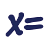Games
Problems
Go Pro!

# Systems of Equations

Reference > Mathematics > AlgebraSolving Systems of equations - an introductory explanation and unit outlineSolving systems of equations in two unknowns using the addition methodSolving systems of equations in two unknowns, in which the terms need to be rearranged to help properly combine themSolving systems of two equations in two unknowns using the substitution methodHow to determine whether a system of equations is indeterminate, or inconsistentSolving word problems which involve two variables involves converting the information into two equationsSolving systems of equations involving fractions, with variables in the numerator - or the denominatorHow to solve a system of three equations in three unknownsLike us on Facebook to get updates about new resources